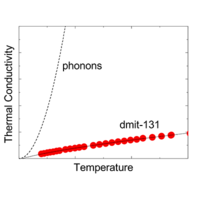Physical Review X ( IF 12.577 ) Pub Date : 2019-12-10 , DOI: 10.1103/physrevx.9.041051
P. Bourgeois-Hope, F. Laliberté, E. Lefrançois, G. Grissonnanche, S. René de Cotret, R. Gordon, S. Kitou, H. Sawa, H. Cui, R. Kato, L. Taillefer, and N. Doiron-LeyraudThe thermal conductivity $\kappa$ of the quasi-2D organic spin-liquid candidate ${\mathrm{EtMe}}_{3}\mathrm{Sb}\left[\mathrm{Pd}\left(\mathrm{dmit}{\right)}_{2}{\right]}_{2}$ (dmit-131) was measured at low temperatures, down to 0.07 K. We observe a vanishingly small residual linear term ${\kappa }_{0}/T$, in $\kappa /T$ vs $T$ as $T\to 0$. This shows that the low-energy excitations responsible for the sizable residual linear term $\gamma$ in the specific heat $C$, seen in $C/T$ vs $T$ as $T\to 0$, are localized. We conclude that there are no mobile gapless excitations in this spin-liquid candidate, in contrast with a prior study of dmit-131 that reported a large ${\kappa }_{0}/T$ value [ , Science 328, 1246 (2010)]. Our study shows that dmit-131 is in fact similar to $\kappa \text{−}\left(\mathrm{BEDT}\text{−}\mathrm{TTF}{\right)}_{2}{\mathrm{Cu}}_{2}\left(\mathrm{CN}{\right)}_{3}$, another quasi-2D organic spin-liquid candidate where a vanishingly small ${\kappa }_{0}/T$ and a sizable $\gamma$ are seen. We attribute heat conduction in these organic insulators without magnetic order to phonons undergoing strong spin-phonon scattering, as observed in several other spin-liquid materials.

down
wechat
bug